# Estimating Times of Second and Third Contacts

The times of second and third contact for any location not listed in this publication can be estimated using the detailed maps found in the final section. Alternatively, the contact times can be estimated from maps on which the umbral path has been plotted. Table 7 lists the path coordinates conveniently arranged in 1° increments of longitude to assist plotting by hand. The path coordinates in Table 3 define a line of maximum eclipse at five minute increments in time. These lines of maximum eclipse each represent the projection diameter of the umbral shadow at the given time. Thus, any point on one of these lines will witness maximum eclipse (i.e.: mid-totality) at the same instant. The coordinates in Table 3 should be added to the map in order to construct lines of maximum eclipse.

The estimation of contact times for any one point begins with an interpolation for the time of maximum eclipse at that location. The time of maximum eclipse is proportional to a point's distance between two adjacent lines of maximum eclipse, measured along a line parallel to the center line. This relationship is valid along most of the path with the exception of the extreme ends, where the shadow experiences its largest acceleration. The center line duration of totality D and the path width W are similarly interpolated from the values of the adjacent lines of maximum eclipse as listed in Table 3. Since the location of interest probably does not lie on the center line, it is useful to have an expression for calculating the duration of totality d as a function of its perpendicular distance a from the center line: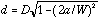where: d = duration of totality at desired location (seconds)

D = duration of totality on the center line (seconds)

a = perpendicular distance from the center line (kilometers)

W = width of the path (kilometers)

If tm is the interpolated time of maximum eclipse for the location, then the approximate times of second and third contacts (t2 and t3, respectively) are:

Second Contact: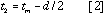Third Contact: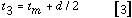The position angles of second and third contact (either P or V) for any location off the center line are also useful in some applications. First, linearly interpolate the center line position angles of second and third contacts from the values of the adjacent lines of maximum eclipse as listed in Table 5. If X2 and X3 are the interpolated center line position angles of second and third contacts, then the position angles x2 and x3 of those contacts for an observer located a kilometers from the center line are:

Second Contact: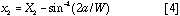Third Contact: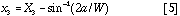where: x_n = interpolated position angle (either P or V) of contact n at location

X_n = interpolated position angle (either P or V) of contact n on center line

a = perpendicular distance from the center line (kilometers)

(use negative values for locations south of the center line)

W = width of the path (kilometers)

Next section